GRE Subject Test: Math : Complex Plane

Example Questions

Example Question #111 : Algebra

Write the complex number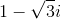in polar form, that is, in terms of a distance from the originon the complex plane and an angle from the positive-axis,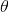, measured in radians.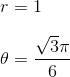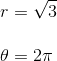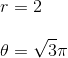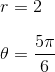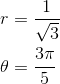Explanation:

To see what the polar form of the number is, it helps to draw it on a graph, where the horizontal axis is the imaginary part and the vertical axis the real part. This is called the complex plane.To find the angle, we can find its supplementary angle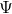and subtract it fromradians, so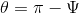.

Using trigonometric ratios,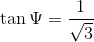and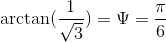.

Then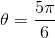.

To find the distance, we need to find the distance from the origin to the point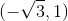. Using the Pythagorean Theorem to find the hypotenuse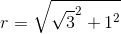or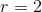.

All GRE Subject Test: Math Resources